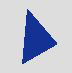Method

# Graphics.DrawPolygon

Warning

This item was deprecated in version 2020r1. Please use Graphics.DrawPath as a replacement.

## Description

Draws a polygon using the values in the 1-based array passed as the X and Y coordinates. The polygon is drawn in the current color. The current color is set with the Graphics.DrawingColor property.

## Sample code

Polygons are drawn using the DrawPolygon and FillPolygon methods of the Graphics class. Polygons are drawn by passing the DrawPolygon or FillPolygon method an Integer array that contains each point in the polygon. This is a 1-based array where odd numbered array elements contain X values and even numbered array elements contain Y coordinates. This means that element 1 contains the X coordinate of the first point in the polygon and element 2 contains the Y coordinate of the first point in the polygon. Consider the following array values:

{| cellpadding="8" cellspacing="0" border="1" ! width=30% style="background-color:#e0e0e0" |Element # ! width=30% style="background-color:#e0e0e0" |Value |- |1 |10 |- |2 |5 |- |3 |40 |- |4 |40 |- |5 |5 |- |6 |60 |}

When passed to the DrawPolygon or FillPolygon method, this array would draw a polygon by drawing a line starting at 10,5 and ending at 40,40 then drawing another line starting from 40,40 ending at 5,60 and finally a line from 5,60 back to 10,5 to complete the polygon. This polygon has only three sets of coordinates so it is a triangle.

The code in the Paint event of a Canvas control or Window to draw this polygon, looks like this. The code is in the Paint event.

```Var points(6) As Double
points(1) = 10
points(2) = 5
points(3) = 40
points(4) = 40
points(5) = 5
points(6) = 60
g.DrawPolygon(points)
```

FillPolygon draws the same polygon but with the interior filled with the ForeColor:Another way of populating the array is with the Array function. The Array function takes a list of values separated by commas and populates the array, beginning with element zero. Since the first element that DrawPolygon uses is element 1, you can use any value in element zero. This code is in the Paint event.

```Var points() As Double
points = Array(0, 10, 5, 40, 40, 5, 60)
g.DrawPolygon(points)
```

## Compatibility

All project types on all supported operating systems.